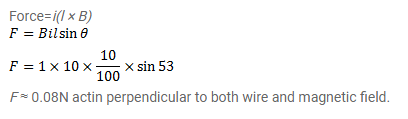# Consider a 10cm long portion of a straight wire carrying

Question:

Consider a $10 \mathrm{~cm}$ long portion of a straight wire carrying a current of $10 \mathrm{~A}$ placed in a magnetic field of $0.1 \mathrm{~T}$ making an angle of $53^{\circ}$ with the wire. What magnetic force does the wire experience?

Solution: